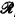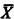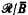Home

# Some theorems on R-maximal sets and major subsets of recursively enumerable sets

## Extract

In , we studied the relational systems/Ā obtained from the recursive functions of one variable by identifying two such functions if they are equal for all but finitely many хĀ, where Ā is an r-cohesive set. The relational systems/Ā with addition and multiplication defined pointwise on them, were once thought to be potential candidates for nonstandard models of arithmetic. This, however, turned out not to be the case, as was shown by Feferman, Scott, and Tennenbaum . We showed, letting A and B be r-maximal sets, and lettingdenote the complement of X, that/Ā andare elementarily equivalent (/Ā) if there are r-maximal supersets C and D of A and B respectively such that C and D have the same many-one degree (C =mD). In fact, if A and B are maximal sets,/Āif, and only if, A =mB. We wish to study the relationship between the elementary equivalence of/Ā and, and the Turing degrees of A and B.

Hide All

# Some theorems on R-maximal sets and major subsets of recursively enumerable sets

## Metrics

### Full text viewsFull text views reflects the number of PDF downloads, PDFs sent to Google Drive, Dropbox and Kindle and HTML full text views.

Total number of HTML views: 0
Total number of PDF views: 0 *Loading metrics...

### Abstract viewsAbstract views reflect the number of visits to the article landing page.

Total abstract views: 0 *Loading metrics...

* Views captured on Cambridge Core between <date>. This data will be updated every 24 hours.

Usage data cannot currently be displayed